Hierarchical cluster analysis on famous data sets - enhanced with the dendextend package

Introduction

This document demonstrates, on several famous data sets, how the dendextend R package can be used to enhance Hierarchical Cluster Analysis (through better visualization and sensitivity analysis).

iris - Edgar Anderson’s Iris Data

Background

The famous (Fisher’s or Anderson’s) iris data set gives the measurements in centimeters of the variables sepal length and width and petal length and width, respectively, for 50 flowers from each of 3 species of iris. The species are Iris setosa, versicolor, and virginica. (from ?iris)

The Iris flower data set is fun for learning supervised classification algorithms, and is known as a difficult case for unsupervised learning. This is easily seen through the following Scatter Plot Matrix (SPLOM):

Define variables:

iris <- datasets::iris
iris2 <- iris[,-5]
species_labels <- iris[,5]
library(colorspace) # get nice colors
species_col <- rev(rainbow_hcl(3))[as.numeric(species_labels)]

SPLOM:

# Plot a SPLOM:
pairs(iris2, col = species_col,
lower.panel = NULL,
cex.labels=2, pch=19, cex = 1.2)

par(xpd = TRUE)
legend(x = 0.05, y = 0.4, cex = 2,
legend = as.character(levels(species_labels)),
fill = unique(species_col))
par(xpd = NA)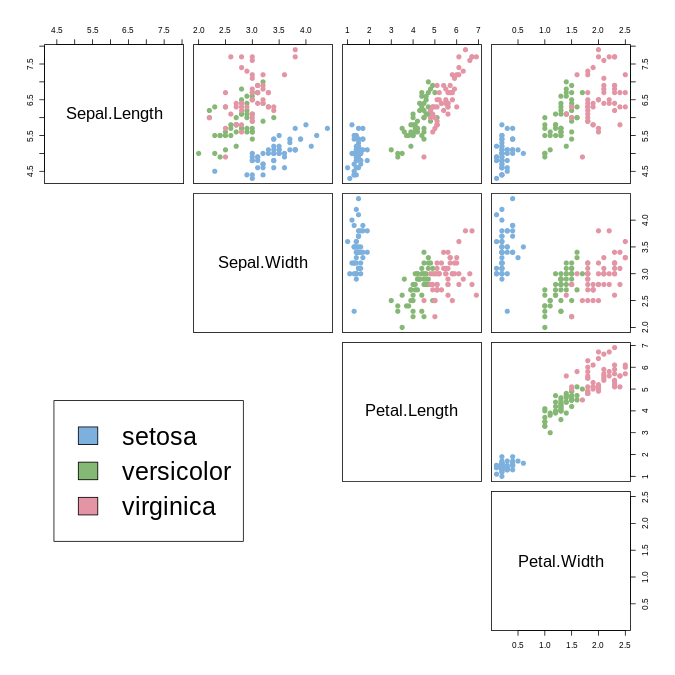We can see that the Setosa species are distinctly different from Versicolor and Virginica (they have lower petal length and width). But Versicolor and Virginica cannot easily be separated based on measurements of their sepal and petal width/length.

The same conclusion can be made by looking at the parallel coordinates plot of the data:

# http://blog.safaribooksonline.com/2014/03/31/mastering-parallel-coordinate-charts-r/
par(las = 1, mar = c(4.5, 3, 3, 2) + 0.1, cex = .8)
MASS::parcoord(iris2, col = species_col, var.label = TRUE, lwd = 2)

title("Parallel coordinates plot of the Iris data")
par(xpd = TRUE)
legend(x = 1.75, y = -.25, cex = 1,
legend = as.character(levels(species_labels)),
fill = unique(species_col), horiz = TRUE)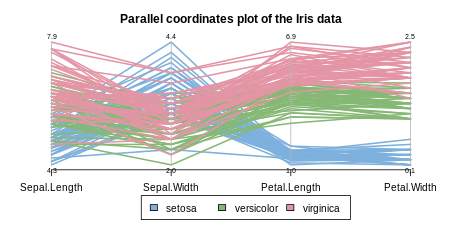par(xpd = NA)

The 3 clusters from the “complete” method vs the real species category

The default hierarchical clustering method in hclust is “complete”. We can visualize the result of running it by turning the object to a dendrogram and making several adjustments to the object, such as: changing the labels, coloring the labels based on the real species category, and coloring the branches based on cutting the tree into three clusters.

d_iris <- dist(iris2) # method="man" # is a bit better
hc_iris <- hclust(d_iris, method = "complete")
iris_species <- rev(levels(iris[,5]))

library(dendextend)
dend <- as.dendrogram(hc_iris)
# order it the closest we can to the order of the observations:
dend <- rotate(dend, 1:150)

# Color the branches based on the clusters:
dend <- color_branches(dend, k=3) #, groupLabels=iris_species)

# Manually match the labels, as much as possible, to the real classification of the flowers:
labels_colors(dend) <-
rainbow_hcl(3)[sort_levels_values(
as.numeric(iris[,5])[order.dendrogram(dend)]
)]

# We shall add the flower type to the labels:
labels(dend) <- paste(as.character(iris[,5])[order.dendrogram(dend)],
"(",labels(dend),")",
sep = "")
# We hang the dendrogram a bit:
dend <- hang.dendrogram(dend,hang_height=0.1)
# reduce the size of the labels:
# dend <- assign_values_to_leaves_nodePar(dend, 0.5, "lab.cex")
dend <- set(dend, "labels_cex", 0.5)
# And plot:
par(mar = c(3,3,3,7))
plot(dend,
main = "Clustered Iris data set
(the labels give the true flower species)",
horiz =  TRUE,  nodePar = list(cex = .007))
legend("topleft", legend = iris_species, fill = rainbow_hcl(3))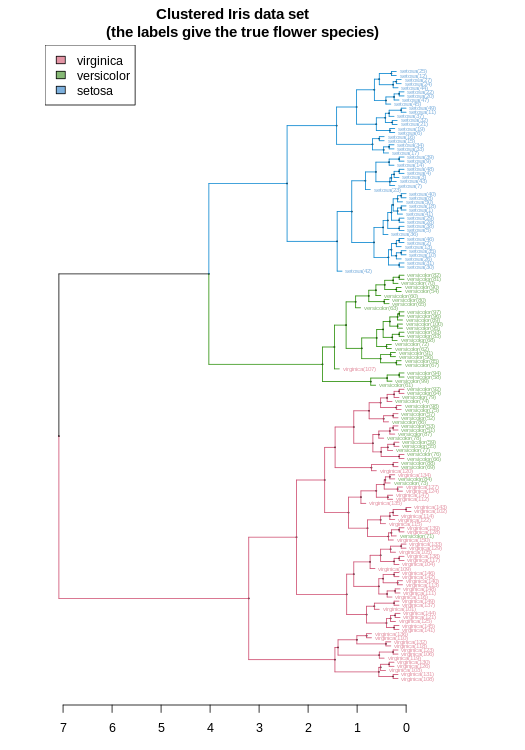#### BTW, notice that:
# labels(hc_iris) # no labels, because "iris" has no row names
# is.integer(labels(dend)) # this could cause problems...
# is.character(labels(dend)) # labels are no longer "integer"

The same can be presented in a circular layout:

# Requires that the circlize package will be installed
par(mar = rep(0,4))
circlize_dendrogram(dend)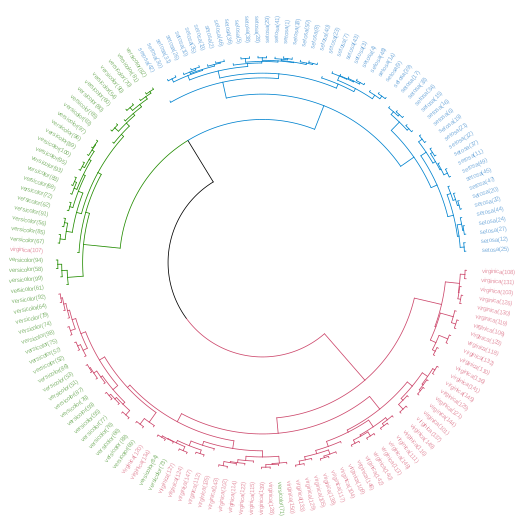These visualizations easily demonstrates how the separation of the hierarchical clustering is very good with the “Setosa” species, but misses in labeling many “Versicolor” species as “Virginica”.

The hanging of the tree also helps to locate extreme observations. For example, we can see that observation “virginica (107)” is not very similar to the Versicolor species, but still, it is among them. Also, “Versicolor (71)” is located too much “within” the group of Virginica flowers.

We can also explore the data using a heatmap. The rows are ordered based on the order of the hierarchical clustering (using the “complete” method). The colored bar indicates the species category each row belongs to. The color in the heatmap indicates the length of each measurement (from light yellow to dark red).

In the heatmap we also see how the Setosa species has low petal values (in light yellow), but it is very difficult to see any clear distinction between the other two species.

some_col_func <- function(n) rev(colorspace::heat_hcl(n, c = c(80, 30), l = c(30, 90), power = c(1/5, 1.5)))

# scaled_iris2 <- iris2 %>% as.matrix %>% scale
# library(gplots)
gplots::heatmap.2(as.matrix(iris2),
main = "Heatmap for the Iris data set",
srtCol = 20,
dendrogram = "row",
Rowv = dend,
Colv = "NA", # this to make sure the columns are not ordered
trace="none",
margins =c(5,0.1),
key.xlab = "Cm",
denscol = "grey",
density.info = "density",
RowSideColors = rev(labels_colors(dend)), # to add nice colored strips
col = some_col_func
)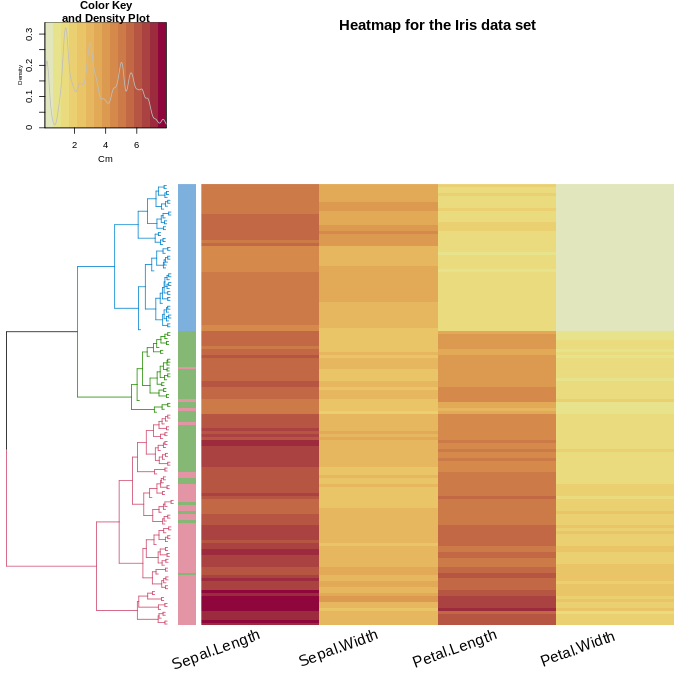We can get an interactive heatmap by using the heatmaply package/function: (code is not evaluated in order to keep the HTML size)

heatmaply::heatmaply(as.matrix(iris2),
dendrogram = "row",
Rowv = dend)

Similarity/difference between various clustering algorithms

We may ask ourselves how many different results we could get if we would use different clustering algorithms (hclust has 8 different algorithms implemented). For the purpose of this analysis, we will create all 8 hclust objects, and chain them together into a single dendlist object (which, as the name implies, can hold a bunch of dendrograms together for the purpose of further analysis).

hclust_methods <- c("ward.D", "single", "complete", "average", "mcquitty",
"median", "centroid", "ward.D2")
iris_dendlist <- dendlist()
for(i in seq_along(hclust_methods)) {
hc_iris <- hclust(d_iris, method = hclust_methods[i])
iris_dendlist <- dendlist(iris_dendlist, as.dendrogram(hc_iris))
}
names(iris_dendlist) <- hclust_methods
iris_dendlist
## \$ward.D
## 'dendrogram' with 2 branches and 150 members total, at height 199.6205
##
## \$single
## 'dendrogram' with 2 branches and 150 members total, at height 1.640122
##
## \$complete
## 'dendrogram' with 2 branches and 150 members total, at height 7.085196
##
## \$average
## 'dendrogram' with 2 branches and 150 members total, at height 4.062683
##
## \$mcquitty
## 'dendrogram' with 2 branches and 150 members total, at height 4.497283
##
## \$median
## 'dendrogram' with 2 branches and 150 members total, at height 2.82744
##
## \$centroid
## 'dendrogram' with 2 branches and 150 members total, at height 2.994307
##
## \$ward.D2
## 'dendrogram' with 2 branches and 150 members total, at height 32.44761
##
## attr(,"class")
##  "dendlist"

Next, we can look at the cophenetic correlation between each clustering result using cor.dendlist. (This can be nicely plotted using the corrplot function from the corrplot package):

iris_dendlist_cor <- cor.dendlist(iris_dendlist)
iris_dendlist_cor
##             ward.D    single  complete   average  mcquitty    median  centroid
## ward.D   1.0000000 0.9836838 0.5774013 0.9841333 0.9641103 0.9451815 0.9809088
## single   0.9836838 1.0000000 0.5665529 0.9681156 0.9329029 0.9444723 0.9903934
## complete 0.5774013 0.5665529 1.0000000 0.6195121 0.6107473 0.6889092 0.5870062
## average  0.9841333 0.9681156 0.6195121 1.0000000 0.9828015 0.9449422 0.9801444
## mcquitty 0.9641103 0.9329029 0.6107473 0.9828015 1.0000000 0.9203374 0.9499123
## median   0.9451815 0.9444723 0.6889092 0.9449422 0.9203374 1.0000000 0.9403569
## centroid 0.9809088 0.9903934 0.5870062 0.9801444 0.9499123 0.9403569 1.0000000
## ward.D2  0.9911648 0.9682507 0.6096286 0.9895131 0.9829977 0.9445832 0.9737886
##            ward.D2
## ward.D   0.9911648
## single   0.9682507
## complete 0.6096286
## average  0.9895131
## mcquitty 0.9829977
## median   0.9445832
## centroid 0.9737886
## ward.D2  1.0000000
corrplot::corrplot(iris_dendlist_cor, "pie", "lower")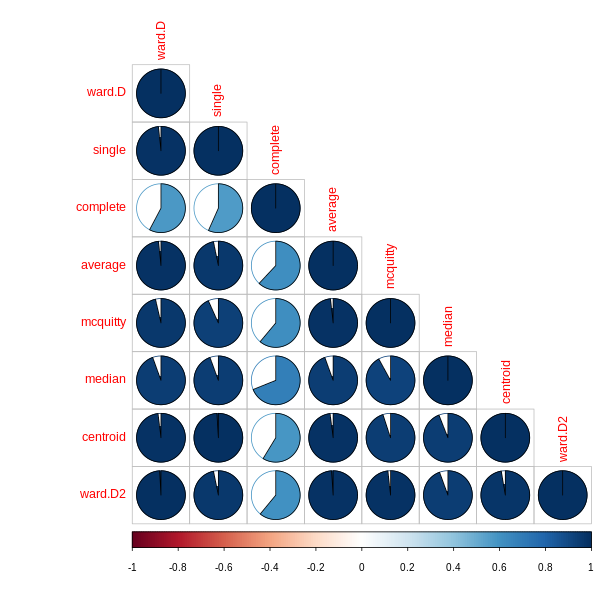From the above figure, we can easily see that most clustering methods yield very similar results, except for the complete method (the default method in hclust), which yields a correlation measure of around 0.6.

The default cophenetic correlation uses pearson’s measure, but what if we use the spearman’s correlation coefficient?

iris_dendlist_cor_spearman <- cor.dendlist(iris_dendlist, method_coef = "spearman")
corrplot::corrplot(iris_dendlist_cor_spearman, "pie", "lower")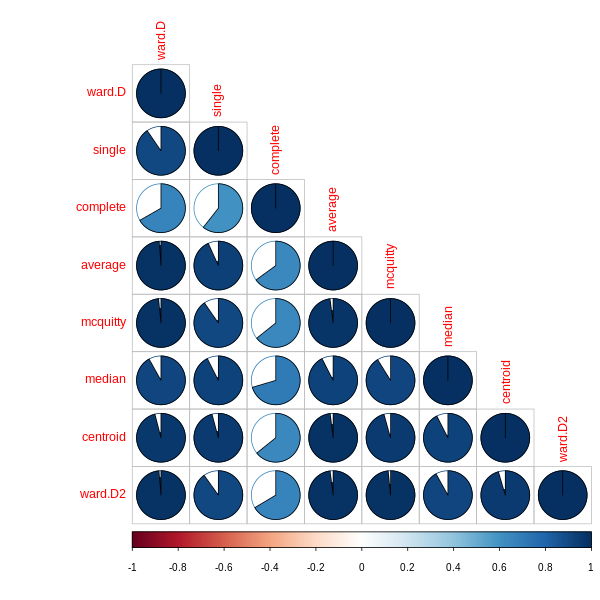We can see that the correlations are not so strong, indicating a behavior that is dependent on some items which are very distant from one another having an influence on the pearson’s correlation more than that of the spearman’s correlation.

To further explore the similarity and difference between the alternative clustering algorithms, we can turn to using the tanglegram function (which works for either two dendrograms, or a dendlist).

First, let us see two methods which are very similar: ward.D vs ward.D2. From a first glance, we can see how they both give the same result for the top 3 clusters. However, since they are both ladderizes (i.e.: having their smaller branch rotated to be higher for each node), we can see that their clustering is not identical (due to the crossings).

# The `which` parameter allows us to pick the elements in the list to compare
iris_dendlist %>% dendlist(which = c(1,8)) %>% ladderize %>%
set("branches_k_color", k=3) %>%
# untangle(method = "step1side", k_seq = 3:20) %>%
# set("clear_branches") %>% #otherwise the single lines are not black, since they retain the previous color from the branches_k_color.
tanglegram(faster = TRUE) # (common_subtrees_color_branches = TRUE)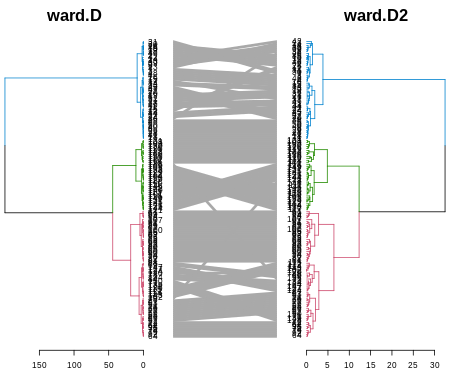Next, let us look at two methods which also have a high cophenetic correlation: ward.D vs the average:

# The `which` parameter allows us to pick the elements in the list to compare
iris_dendlist %>% dendlist(which = c(1,4)) %>% ladderize %>%
set("branches_k_color", k=2) %>%
# untangle(method = "step1side", k_seq = 3:20) %>%
tanglegram(faster = TRUE) # (common_subtrees_color_branches = TRUE)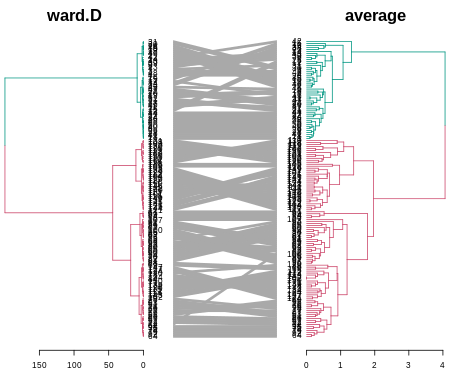We see that when it comes to the major clusters, the two algorithms perform quite similarly.

However, how are they doing inside each of the clusters? It is quite difficult to compare the two because of the high value in ward.D. For comparison purposes, we can “rank” the heights of the branches in the two dendrograms (while still preserving their internal order). Next, we can highlight the shared common sub-trees (with different colors), and the distinct edges (with a dashed line):

# The `which` parameter allows us to pick the elements in the list to compare
iris_dendlist %>% dendlist(which = c(1,4)) %>% ladderize %>%
# untangle(method = "step1side", k_seq = 3:20) %>%
set("rank_branches") %>%
tanglegram(common_subtrees_color_branches = TRUE)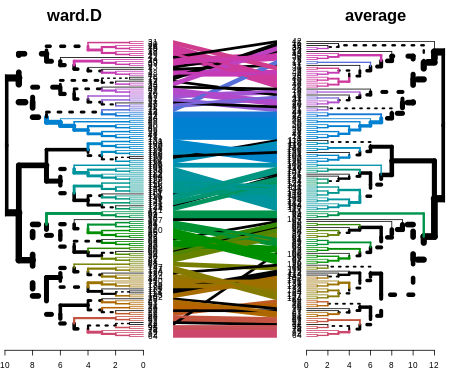We have 39 sub-trees that are identical between the two dendrograms:

length(unique(common_subtrees_clusters(iris_dendlist[], iris_dendlist[]))[-1])
##  39
# -1 at the end is because we are ignoring the "0" subtree, which indicates leaves that are singletons.

What we can learn from this is that actually the two algorithms seem to give quite different results in the high resolution (higher cuts). However, since both capture the two major clusters (Setosa vs the others), they are considered quite similar by the cophenetic correlation.

But what about the “complete” method (that got a lower cophenetic correlation than the other methods)? When we compare “complete” vs “average”, we can quickly see that in the “complete” method, the splitting of the clusters is much more balanced, and mixes the “Setosa” species with another one. This is probably the cause for the big difference found in the cophenetic correlation between the “complete method” and the other clustering methods:

iris_dendlist %>% dendlist(which = c(3,4)) %>% ladderize %>%
untangle(method = "step1side", k_seq = 2:6) %>%
set("branches_k_color", k=2) %>%
tanglegram(faster = TRUE) # (common_subtrees_color_branches = TRUE)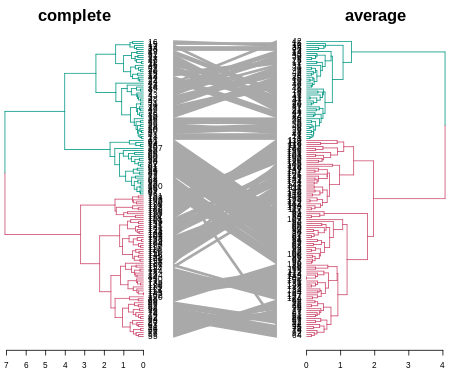We can quickly plot all 8 methods to see this phenomenon (i.e.: that “complete” has its smaller cluster larger than it is in all the other clustering methods):

par(mfrow = c(4,2))
for(i in 1:8) {
iris_dendlist[[i]] %>% set("branches_k_color", k=2) %>% plot(axes = FALSE, horiz = TRUE)
title(names(iris_dendlist)[i])
}